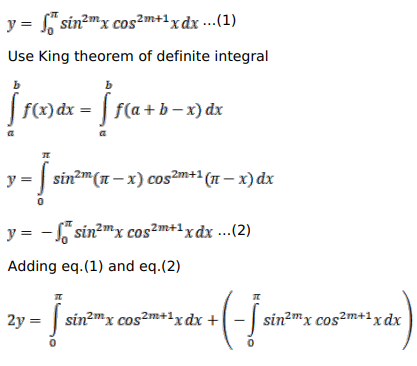# Solve this followingQuestion:

Prove that

$\int_{0}^{\pi} \sin ^{2 m} x \cos ^{2 m+1} x d x=0$, where $m$ is a positive integer

Solution:$y=0$